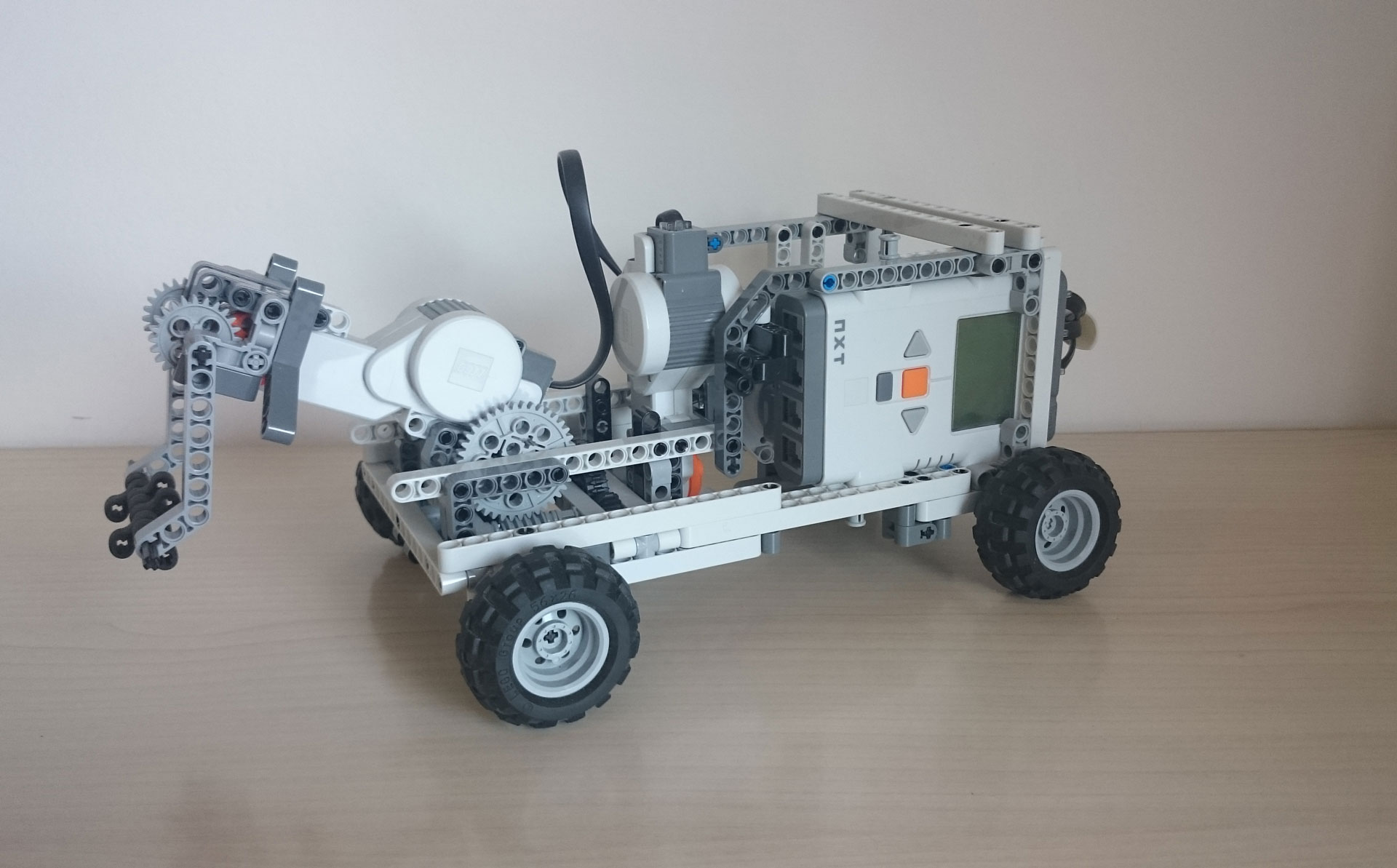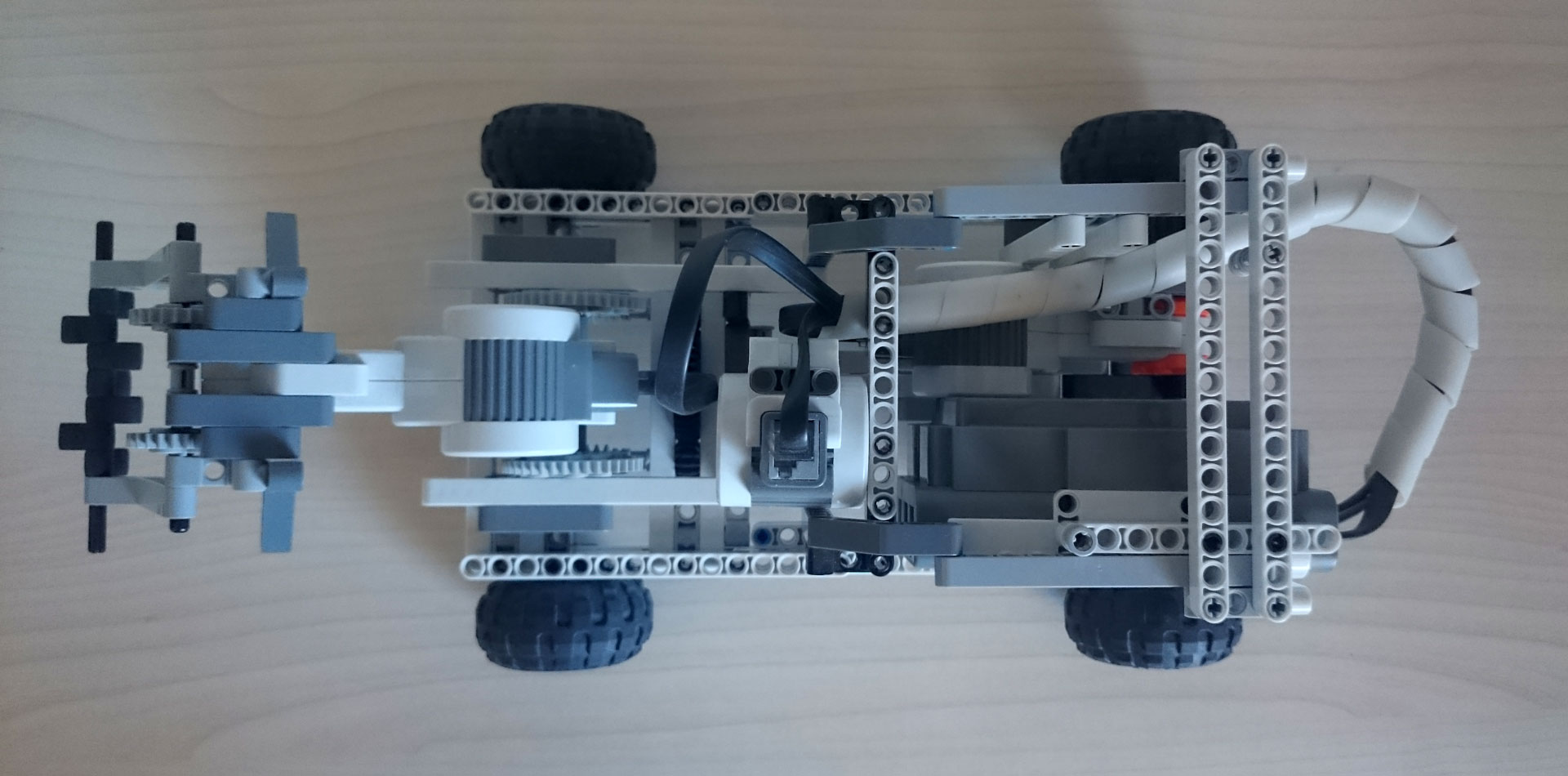In machine learning problems, it is often impossible to provide training examples to teach a software agent how to behave in a particular environment. Actually, in some cases, the environment is partially unknown: a specific set of correct and exhaustive input/output pairs can not be determined. In such situations, supervised learning is not feasible because no model of correct behavior is available: a different, model-free approach has to be adopted.
Reinforcement learning uses an environmental value called reward, which has to be maximized over time. With this assumption, all the problems of this type can be solved with a function to be optimized. The software attemps to determine a mapping between states of the machine and the actions that have to be taken to maximize the reward. This is called policy. When the the optimization algorithm converges, only a sequence of actions (optimal policy) is considered and this is the best in increasing the reward.
Q-learning is one of these algorithms and attemps to find the optimal policy using a matrix of pairs between action and states.

# The agentThe software is implemented on a LEGO Mindstorms NXT in NXC language, which provides a good flexibility and a low level access to the hardware resources. Our agent is a single armed robot which has to learn how to move its arm to crawl. The feedback of its actions is acquired through the encoders in the servo motor connected to the wheels.
The arm is composed by two joints and two links: arm and hand. The hand has a free extremity, instead the arm is connected to the robot.
To provide better stability and improved torque, some reduction gears have been applied between motors and connected links.
Building instructions can be found here.

For some reason two worm gears are missing in the instructions. It can be figured out which ones they are because without them the arm can not be moved.

Top view of the robot:# Q-learning

This algorithm has three main assumptions:

• a model of the environment is known
• the complete set of doable actions is known
• an environmental value used as reward is available

## Model of the environment

In this particular case the robot acts on itself, thus the agent can be considered the environment. In particular it is necessary to define what are the possible states in which the agent can be. In the case of a path finding agent, the states are the possibile positions of the agent in the environment. Instead, for this robot, the states are all the possible position configurations that the arm and the hand can have.
At first glance, the number of total states is the cartesian product of hand states and arm states, nevertheless, arm and hand states are infinite, and the consequence is an infinite number of total states. The solution is to discretize arm and hand positions, for example using a step of 30°. Moreover, both the arm and the hand can not rotate by 360°: the total range, which is smaller, can be measured using the sensor functionality of NXT motors.
For example 4 states will be used for both the hand and the arm: the total number of states is 16. The position of each link will be encoded with a number between 0 and 3.

## Set of actions

Given the structure of the agent, only two types of actions can be done for each joint: rotate in a sense or in the other. In conclusion there are 4 overall actions doable by the robot:

The definition of NONE action is important because it is used for the initialization of the vector containing the sequence of actions.

## Reward

The reward is the movement of the robot: it is measured through the wheels. Also, velocity can be used, but it is required to compute the ratio between the displacement of the robot and the number of iterations.

## Algorithm structure

The algorithm starts with the initialization of all the variables:

• A matrix Q with dimension equal to the cartesian product between the number of states and the number of actions: its value measures the consequences in terms of reward of each action for a given state.
• A matrix E (eligibility) with the same dimension of Q: it is used to create a policy and makes Q higher for actions leading to a greater reward in successive steps.

Both Q and E matrices should be initialized with zeros.

• Two vectors to store actions

The two vectors of actions should be initialized with action NONE (-1);

The complete initialization code is the following:

The optimization part consists in a cycle which termines only when the sequence of actions is optimal, i.e. when it is the same of the previous cycle. The cycle is composed by the following steps:

• Between all the possible actions doable in the current state choose the one with the highest Q, if they all have the same value, then do a random action.
• Do the action and get the reward.
• Update the sequence vector and the matrices.
• If the reward is positive check the convergence of the sequence of actions:
• if convergency happens then exit
• otherwise reset the agent to initial position
• else do another action.

## Choosing the action

The choice of the action to do is based on two opposite approaches: exploitation and exploration.

• Exploitation means to use the acquired knowledge of the environment to choose an action which maximizes the reward: it is a reasoned choice.
• Exploration means to choose arbitrarily the following action.

There are many criteria to select the right approach: in this case the exploration behavior is used only when acquired knowledge is missing or it is unuseful.
For example, a probabilistic approach could have been applied: the kind of approach adopted depends on the result of a random outcome.

## Actions execution

Executing the action is a matter of motor rotation, with some simple lines of code it is easily achieved. It is important to save the motor position before and after the action to compute the value of the reward.

## Updating matrices

The core of the algorithm is made by Q and E matrices.
In general, for each value of the matrices
$$Q_{i+1}= (1-\alpha) \cdot Q_i+\alpha\cdot\delta\cdot E_i$$

$$E_{i+1}=E_i \cdot \tau$$

where
$$\delta = (reward + (\beta \cdot Q_{MAX})-Q_P)$$

• QP is the Q value of the old state and the current action.
• reward does not need any explanation.
• QMAX is the best Q available for the current state considering all the possible actions. It is not important to filter only available actions in particular states, because the actions that are not possible to be done will have always a 0 value for Q as they can not be chosen.
• α, β, τ are coefficients between 0 and 1 and they tune the algorithm. In particular
• α is the rate of learning: an higher value will produce a faster learning behavior
• β is the weight of the policy (farsight): the higher it is, the more the following possible actions will influence the choice of the current action
• τ is the forgetting factor

Before the computation of Q and E it is necessary to update the eligibility value of the action done:
$$E_P = E_P + 1$$EP is the eligibility of the previous state and the current action.

## The main cycle

All these functions have to be used in the main cycle as described before: the following is an example of main function.

# Conclusion

The complete code can be found in my GitHub repo, it requires some parameter tuning. The learning is extremely slow, because the machine is intrinsically slow: if you try to simulate the agent with only software you can see results in short time. For code compilation and deployment, BricxCC is a good choice if you are on Windows, otherwise NBC is available for all platforms, but without IDE.